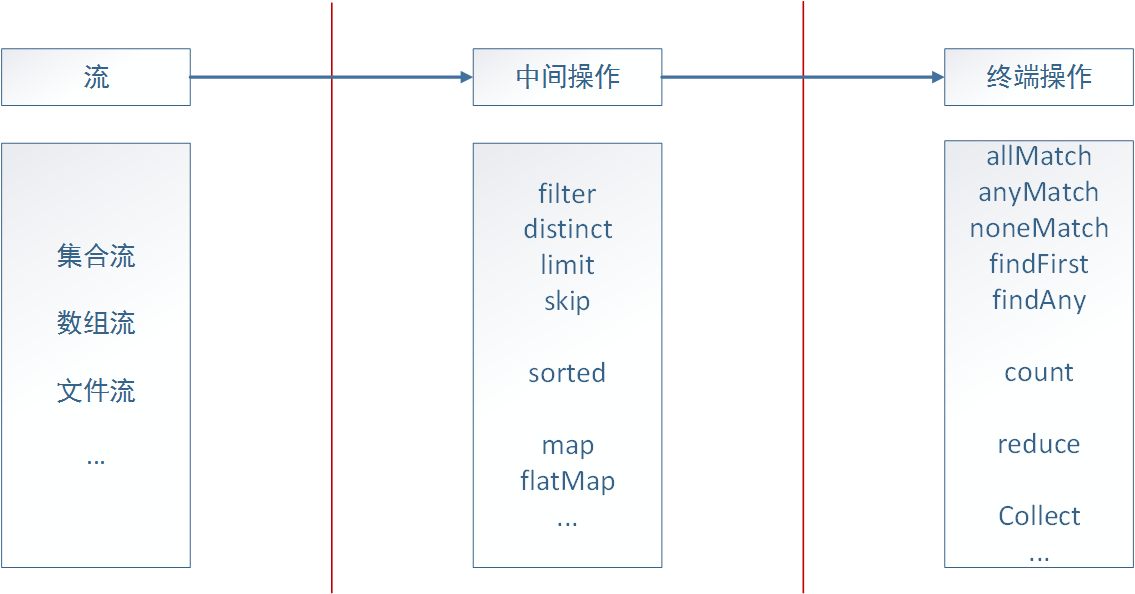Java 8th 新特性系列

### 一. 流式处理简介

在我接触到java8流式数据处理的时候，我的第一感觉是流式处理让集合操作变得简洁了许多，通常我们需要多行代码才能完成的操作，借助于流式处理可以在一行中实现。比如我们希望对一个包含整数的集合中筛选出所有的偶数，并将其封装成为一个新的List返回，那么在java8之前，我们需要通过如下代码实现：

``````List<Integer> evens = new ArrayList<>();
for (final Integer num : nums) {
if (num % 2 == 0) {
}
}
``````

``````List<Integer> evens = nums.stream().filter(num -> num % 2 == 0).collect(Collectors.toList());
``````

先简单解释一下上面这行语句的含义，`stream()`操作将集合转换成一个流，`filter()`执行我们自定义的筛选处理，这里是通过lambda表达式筛选出所有偶数，最后我们通过`collect()`对结果进行封装处理，并通过`Collectors.toList()`指定其封装成为一个List集合返回。
由上面的例子可以看出，java8的流式处理极大的简化了对于集合的操作，实际上不光是集合，包括数组、文件等，只要是可以转换成流，我们都可以借助流式处理，类似于我们写SQL语句一样对其进行操作。java8通过内部迭代来实现对流的处理，一个流式处理可以分为三个部分：转换成流、中间操作、终端操作。如下图：### 二. 中间操作

``````public class Student {

/** 学号 */
private long id;

private String name;

private int age;

/** 年级 */

/** 专业 */
private String major;

/** 学校 */
private String school;

// 省略getter和setter
}
``````
``````// 初始化
List<Student> students = new ArrayList<Student>() {
{
add(new Student(20160001, "孔明", 20, 1, "土木工程", "武汉大学"));
add(new Student(20160002, "伯约", 21, 2, "信息安全", "武汉大学"));
add(new Student(20160003, "玄德", 22, 3, "经济管理", "武汉大学"));
add(new Student(20160004, "云长", 21, 2, "信息安全", "武汉大学"));
add(new Student(20161001, "翼德", 21, 2, "机械与自动化", "华中科技大学"));
add(new Student(20161002, "元直", 23, 4, "土木工程", "华中科技大学"));
add(new Student(20161003, "奉孝", 23, 4, "计算机科学", "华中科技大学"));
add(new Student(20162001, "仲谋", 22, 3, "土木工程", "浙江大学"));
add(new Student(20162002, "鲁肃", 23, 4, "计算机科学", "浙江大学"));
add(new Student(20163001, "丁奉", 24, 5, "土木工程", "南京大学"));
}
};
``````

#### 2.1 过滤

过滤，顾名思义就是按照给定的要求对集合进行筛选满足条件的元素，java8提供的筛选操作包括：filter、distinct、limit、skip。

• filter

在前面的例子中我们已经演示了如何使用filter，其定义为：`Stream<T> filter(Predicate<? super T> predicate)`，filter接受一个谓词`Predicate`，我们可以通过这个谓词定义筛选条件，在介绍lambda表达式时我们介绍过`Predicate`是一个函数式接口，其包含一个`test(T t)`方法，该方法返回`boolean`。现在我们希望从集合`students`中筛选出所有武汉大学的学生，那么我们可以通过filter来实现，并将筛选操作作为参数传递给filter：

``````List<Student> whuStudents = students.stream()
.filter(student -> "武汉大学".equals(student.getSchool()))
.collect(Collectors.toList());
``````
• distinct

distinct操作类似于我们在写SQL语句时，添加的`DISTINCT`关键字，用于去重处理，distinct基于`Object.equals(Object)`实现，回到最开始的例子，假设我们希望筛选出所有不重复的偶数，那么可以添加distinct操作：

``````List<Integer> evens = nums.stream()
.filter(num -> num % 2 == 0).distinct()
.collect(Collectors.toList());
``````
• limit

limit操作也类似于SQL语句中的`LIMIT`关键字，不过相对功能较弱，limit返回包含前n个元素的流，当集合大小小于n时，则返回实际长度，比如下面的例子返回前两个专业为`土木工程`专业的学生：

``````List<Student> civilStudents = students.stream()
.filter(student -> "土木工程".equals(student.getMajor())).limit(2)
.collect(Collectors.toList());
``````

说到limit，不得不提及一下另外一个流操作：`sorted`。该操作用于对流中元素进行排序，sorted要求待比较的元素必须实现`Comparable`接口，如果没有实现也不要紧，我们可以将比较器作为参数传递给`sorted(Comparator<? super T> comparator)`，比如我们希望筛选出专业为土木工程的学生，并按年龄从小到大排序，筛选出年龄最小的两个学生，那么可以实现为：

``````List<Student> sortedCivilStudents = students.stream()
.filter(student -> "土木工程".equals(student.getMajor())).sorted((s1, s2) -> s1.getAge() - s2.getAge())
.limit(2)
.collect(Collectors.toList());
``````
• skip

skip操作与limit操作相反，如同其字面意思一样，是跳过前n个元素，比如我们希望找出排序在2之后的土木工程专业的学生，那么可以实现为：

``````List<Student> civilStudents = students.stream()
.filter(student -> "土木工程".equals(student.getMajor()))
.skip(2)
.collect(Collectors.toList());
``````

通过skip，就会跳过前面两个元素，返回由后面所有元素构造的流，如果n大于满足条件的集合的长度，则会返回一个空的集合。

#### 2.2 映射

在SQL中，借助`SELECT`关键字后面添加需要的字段名称，可以仅输出我们需要的字段数据，而流式处理的映射操作也是实现这一目的，在java8的流式处理中，主要包含两类映射操作：map和flatMap。

• map

举例说明，假设我们希望筛选出所有专业为计算机科学的学生姓名，那么我们可以在filter筛选的基础之上，通过map将学生实体映射成为学生姓名字符串，具体实现如下：

``````List<String> names = students.stream()
.filter(student -> "计算机科学".equals(student.getMajor()))
.map(Student::getName).collect(Collectors.toList());
``````

除了上面这类基础的map，java8还提供了`mapToDouble(ToDoubleFunction<? super T> mapper)``mapToInt(ToIntFunction<? super T> mapper) ``mapToLong(ToLongFunction<? super T> mapper) `，这些映射分别返回对应类型的流，java8为这些流设定了一些特殊的操作，比如我们希望计算所有专业为计算机科学学生的年龄之和，那么我们可以实现如下：

``````int totalAge = students.stream()
.filter(student -> "计算机科学".equals(student.getMajor()))
.mapToInt(Student::getAge).sum();
``````

通过将Student按照年龄直接映射为`IntStream`，我们可以直接调用提供的`sum()`方法来达到目的，此外使用这些数值流的好处还在于可以避免jvm装箱操作所带来的性能消耗。

• flatMap

flatMap与map的区别在于 flatMap是将一个流中的每个值都转成一个个流，然后再将这些流扁平化成为一个流 。举例说明，假设我们有一个字符串数组`String[] strs = {"java8", "is", "easy", "to", "use"};`，我们希望输出构成这一数组的所有非重复字符，那么我们可能首先会想到如下实现：

``````List<String[]> distinctStrs = Arrays.stream(strs)
.map(str -> str.split(""))  // 映射成为Stream<String[]>
.distinct()
.collect(Collectors.toList());
``````

在执行map操作以后，我们得到是一个包含多个字符串（构成一个字符串的字符数组）的流，此时执行distinct操作是基于在这些字符串数组之间的对比，所以达不到我们希望的目的，此时的输出为：

``````[j, a, v, a, 8]
[i, s]
[e, a, s, y]
[t, o]
[u, s, e]
``````

distinct只有对于一个包含多个字符的流进行操作才能达到我们的目的，即对`Stream<String>`进行操作。此时flatMap就可以达到我们的目的：

``````List<String> distinctStrs = Arrays.stream(strs)
.map(str -> str.split(""))  // 映射成为Stream<String[]>
.flatMap(Arrays::stream)  // 扁平化为Stream<String>
.distinct()
.collect(Collectors.toList());
``````

flatMap将由map映射得到的`Stream<String[]>`，转换成由各个字符串数组映射成的流`Stream<String>`，再将这些小的流扁平化成为一个由所有字符串构成的大流`Steam<String>`，从而能够达到我们的目的。
与map类似，flatMap也提供了针对特定类型的映射操作：`flatMapToDouble(Function<? super T,? extends DoubleStream> mapper) ``flatMapToInt(Function<? super T,? extends IntStream> mapper)``flatMapToLong(Function<? super T,? extends LongStream> mapper)`

### 三. 终端操作

终端操作是流式处理的最后一步，我们可以在终端操作中实现对流查找、归约等操作。

#### 3.1 查找

• allMatch

allMatch用于检测是否全部都满足指定的参数行为，如果全部满足则返回true，例如我们希望检测是否所有的学生都已满18周岁，那么可以实现为：

``````boolean isAdult = students.stream().allMatch(student -> student.getAge() >= 18);
``````
• anyMatch

anyMatch则是检测是否存在一个或多个满足指定的参数行为，如果满足则返回true，例如我们希望检测是否有来自武汉大学的学生，那么可以实现为：

``````boolean hasWhu = students.stream().anyMatch(student -> "武汉大学".equals(student.getSchool()));
``````
• noneMatch

noneMatch用于检测是否不存在满足指定行为的元素，如果不存在则返回true，例如我们希望检测是否不存在专业为计算机科学的学生，可以实现如下：

``````boolean noneCs = students.stream().noneMatch(student -> "计算机科学".equals(student.getMajor()));
``````
• findFirst

findFirst用于返回满足条件的第一个元素，比如我们希望选出专业为土木工程的排在第一个学生，那么可以实现如下：

``````Optional<Student> optStu = students.stream().filter(student -> "土木工程".equals(student.getMajor())).findFirst();
``````

findFirst不携带参数，具体的查找条件可以通过filter设置，此外我们可以发现findFirst返回的是一个Optional类型，关于该类型的具体讲解可以参考上一篇：Java8新特性 - Optional类

• findAny

findAny相对于findFirst的区别在于，findAny不一定返回第一个，而是返回任意一个，比如我们希望返回任意一个专业为土木工程的学生，可以实现如下：

``````Optional<Student> optStu = students.stream().filter(student -> "土木工程".equals(student.getMajor())).findAny();
``````

实际上对于顺序流式处理而言，findFirst和findAny返回的结果是一样的，至于为什么会这样设计，是因为在下一篇我们介绍的 并行流式处理，当我们启用并行流式处理的时候，查找第一个元素往往会有很多限制，如果不是特别需求，在并行流式处理中使用findAny的性能要比findFirst好。

#### 3.2 归约

前面的例子中我们大部分都是通过`collect(Collectors.toList())`对数据封装返回，如我的目标不是返回一个新的集合，而是希望对经过参数化操作后的集合进行进一步的运算，那么我们可用对集合实施归约操作。java8的流式处理提供了`reduce`方法来达到这一目的。
前面我们通过mapToInt将`Stream<Student>`映射成为`IntStream`，并通过`IntStream`的sum方法求得所有学生的年龄之和，实际上我们通过归约操作，也可以达到这一目的，实现如下：

``````// 前面例子中的方法
int totalAge = students.stream()
.filter(student -> "计算机科学".equals(student.getMajor()))
.mapToInt(Student::getAge).sum();
// 归约操作
int totalAge = students.stream()
.filter(student -> "计算机科学".equals(student.getMajor()))
.map(Student::getAge)
.reduce(0, (a, b) -> a + b);

// 进一步简化
int totalAge2 = students.stream()
.filter(student -> "计算机科学".equals(student.getMajor()))
.map(Student::getAge)
.reduce(0, Integer::sum);

// 采用无初始值的重载版本，需要注意返回Optional
Optional<Integer> totalAge = students.stream()
.filter(student -> "计算机科学".equals(student.getMajor()))
.map(Student::getAge)
.reduce(Integer::sum);  // 去掉初始值
``````

#### 3.3 收集

前面利用`collect(Collectors.toList())`是一个简单的收集操作，是对处理结果的封装，对应的还有`toSet``toMap`，以满足我们对于结果组织的需求。这些方法均来自于`java.util.stream.Collectors`，我们可以称之为收集器。

##### 3.3.1 归约

收集器也提供了相应的归约操作，但是与reduce在内部实现上是有区别的，收集器更加适用于可变容器上的归约操作，这些收集器广义上均基于`Collectors.reducing()`实现。

• 例1：求学生的总人数
``````long count = students.stream().collect(Collectors.counting());

// 进一步简化
long count = students.stream().count();
``````
• 例2：求年龄的最大值和最小值
``````// 求最大年龄
Optional<Student> olderStudent = students.stream().collect(Collectors.maxBy((s1, s2) -> s1.getAge() - s2.getAge()));

// 进一步简化
Optional<Student> olderStudent2 = students.stream().collect(Collectors.maxBy(Comparator.comparing(Student::getAge)));

// 求最小年龄
Optional<Student> olderStudent3 = students.stream().collect(Collectors.minBy(Comparator.comparing(Student::getAge)));
``````
• 例3：求年龄总和
``````int totalAge4 = students.stream().collect(Collectors.summingInt(Student::getAge));
``````

• 例4：求年龄的平均值
``````double avgAge = students.stream().collect(Collectors.averagingInt(Student::getAge));
``````

• 例5：一次性得到元素个数、总和、均值、最大值、最小值
``````IntSummaryStatistics statistics = students.stream().collect(Collectors.summarizingInt(Student::getAge));
``````

``````IntSummaryStatistics{count=10, sum=220, min=20, average=22.000000, max=24}
``````

• 例6：字符串拼接
``````String names = students.stream().map(Student::getName).collect(Collectors.joining());
// 输出：孔明伯约玄德云长翼德元直奉孝仲谋鲁肃丁奉
String names = students.stream().map(Student::getName).collect(Collectors.joining(", "));
// 输出：孔明, 伯约, 玄德, 云长, 翼德, 元直, 奉孝, 仲谋, 鲁肃, 丁奉
``````
##### 3.3.2 分组

在数据库操作中，我们可以通过`GROUP BY`关键字对查询到的数据进行分组，java8的流式处理也为我们提供了这样的功能`Collectors.groupingBy`来操作集合。比如我们可以按学校对上面的学生进行分组：

``````Map<String, List<Student>> groups = students.stream().collect(Collectors.groupingBy(Student::getSchool));
``````

`groupingBy`接收一个分类器`Function<? super T, ? extends K> classifier`，我们可以自定义分类器来实现需要的分类效果。
上面演示的是一级分组，我们还可以定义多个分类器实现 多级分组，比如我们希望在按学校分组的基础之上再按照专业进行分组，实现如下：

``````Map<String, Map<String, List<Student>>> groups2 = students.stream().collect(
Collectors.groupingBy(Student::getSchool,  // 一级分组，按学校
Collectors.groupingBy(Student::getMajor)));  // 二级分组，按专业
``````

实际上在`groupingBy`的第二个参数不是只能传递groupingBy，还可以传递任意`Collector`类型，比如我们可以传递一个`Collector.counting`，用以统计每个组的个数：

``````Map<String, Long> groups = students.stream().collect(Collectors.groupingBy(Student::getSchool, Collectors.counting()));
``````

##### 3.3.3 分区

分区可以看做是分组的一种特殊情况，在分区中key只有两种情况：true或false，目的是将待分区集合按照条件一分为二，java8的流式处理利用`ollectors.partitioningBy()`方法实现分区，该方法接收一个谓词，例如我们希望将学生分为武大学生和非武大学生，那么可以实现如下：

``````Map<Boolean, List<Student>> partition = students.stream().collect(Collectors.partitioningBy(student -> "武汉大学".equals(student.getSchool())));
``````

分区相对分组的优势在于，我们可以同时得到两类结果，在一些应用场景下可以一步得到我们需要的所有结果，比如将数组分为奇数和偶数。

以上介绍的所有收集器均实现自接口`java.util.stream.Collector`，该接口的定义如下：

``````public interface Collector<T, A, R> {
/**
* A function that creates and returns a new mutable result container.
*
* @return a function which returns a new, mutable result container
*/
Supplier<A> supplier();

/**
* A function that folds a value into a mutable result container.
*
* @return a function which folds a value into a mutable result container
*/
BiConsumer<A, T> accumulator();

/**
* A function that accepts two partial results and merges them.  The
* combiner function may fold state from one argument into the other and
* return that, or may return a new result container.
*
* @return a function which combines two partial results into a combined
* result
*/
BinaryOperator<A> combiner();

/**
* Perform the final transformation from the intermediate accumulation type
* {@code A} to the final result type {@code R}.
*
* <p>If the characteristic {@code IDENTITY_TRANSFORM} is
* set, this function may be presumed to be an identity transform with an
* unchecked cast from {@code A} to {@code R}.
*
* @return a function which transforms the intermediate result to the final
* result
*/
Function<A, R> finisher();

/**
* Returns a {@code Set} of {@code Collector.Characteristics} indicating
* the characteristics of this Collector.  This set should be immutable.
*
* @return an immutable set of collector characteristics
*/
Set<Characteristics> characteristics();

}
``````

我们也可以实现该接口来定义自己的收集器，此处不再展开。

### 四. 并行流式数据处理

流式处理中的很多都适合采用 分而治之 的思想，从而在处理集合较大时，极大的提高代码的性能，java8的设计者也看到了这一点，所以提供了 并行流式处理。上面的例子中我们都是调用`stream()`方法来启动流式处理，java8还提供了`parallelStream()`来启动并行流式处理，`parallelStream()`本质上基于java7的Fork-Join框架实现，其默认的线程数为宿主机的内核数。
启动并行流式处理虽然简单，只需要将`stream()`替换成`parallelStream()`即可，但既然是并行，就会涉及到多线程安全问题，所以在启用之前要先确认并行是否值得（并行的效率不一定高于顺序执行），另外就是要保证线程安全。此两项无法保证，那么并行毫无意义，毕竟结果比速度更加重要，以后有时间再来详细分析一下并行流式数据处理的具体实现和最佳实践。

### 参考文献：